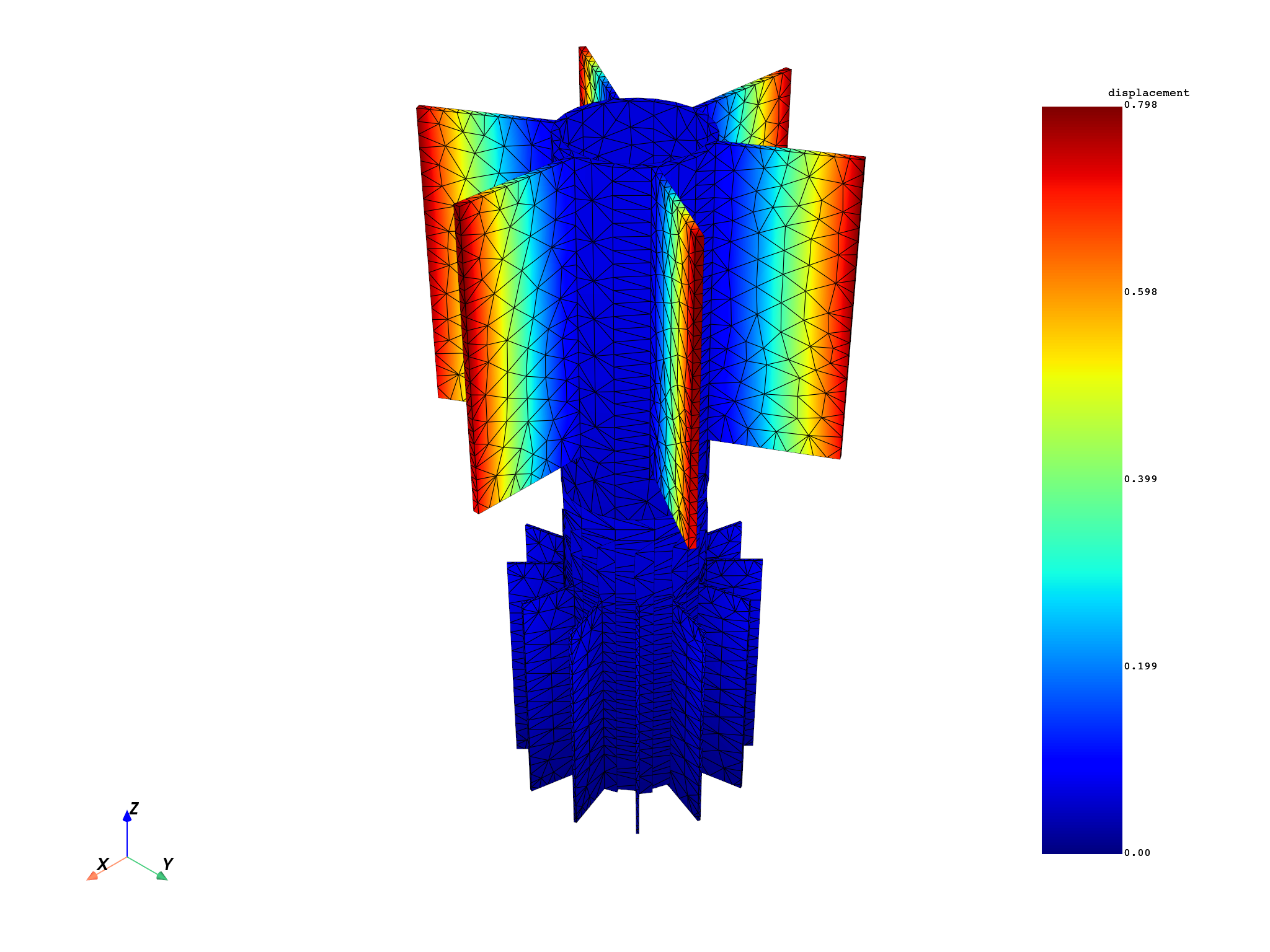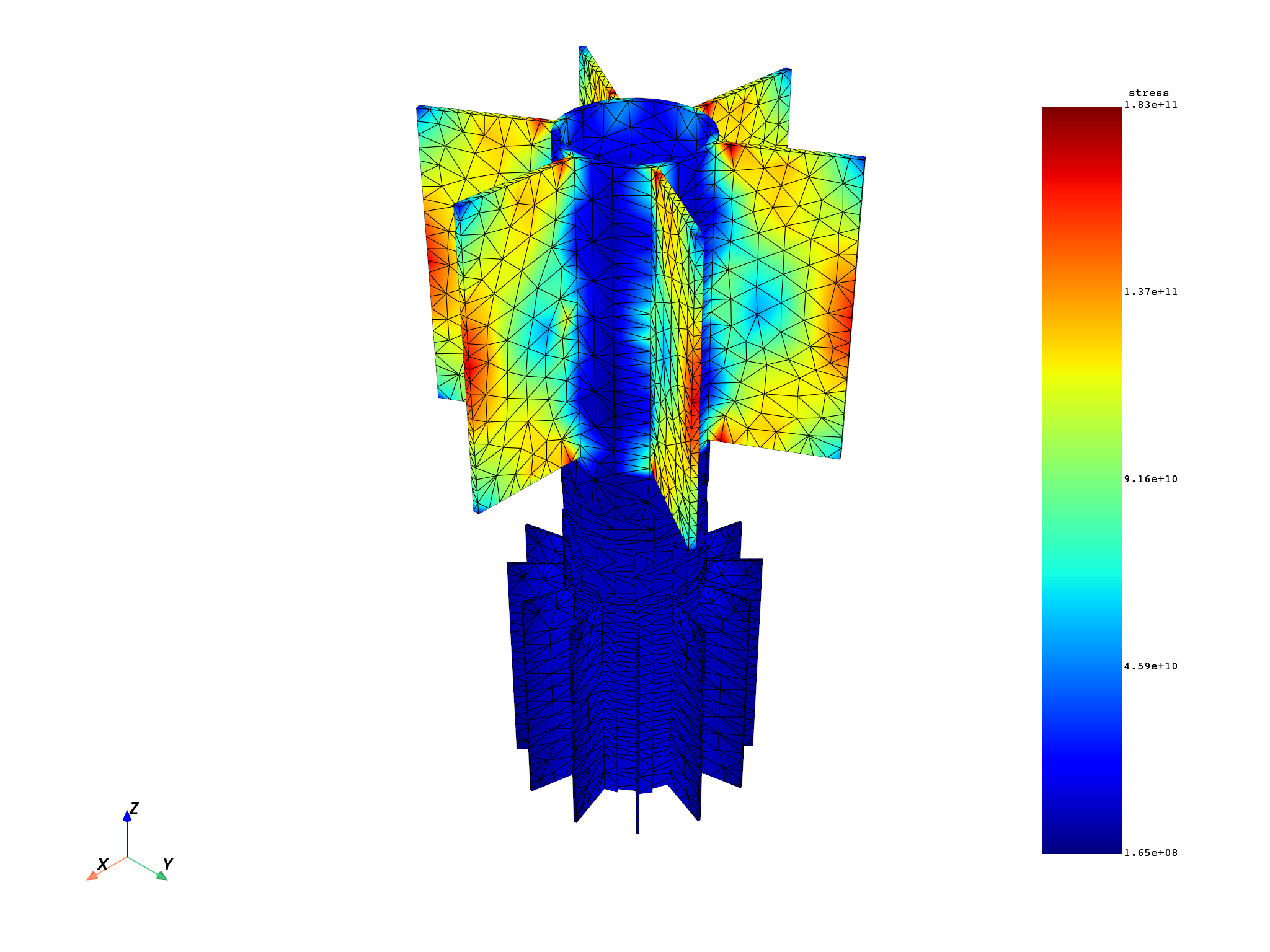# Multi-stage cyclic symmetry example#

This example shows how to expand the mesh and results from a multi-stage cyclic analysis.

```from ansys.dpf import core as dpf
from ansys.dpf.core import examples
```

Create the model and display the state of the result.

```cyc = examples.download_multi_stage_cyclic_result()
model = dpf.Model(cyc)
print(model)
```
```DPF Model
------------------------------
Modal analysis
Unit system: MKS: m, kg, N, s, V, A, degC
Physics Type: Mechanical
Available results:
-  displacement: Nodal Displacement
-  stress: ElementalNodal Stress
-  elastic_strain: ElementalNodal Strain
-  structural_temperature: ElementalNodal Structural temperature
------------------------------
DPF  Meshed Region:
3595 nodes
1557 elements
Unit: m
With solid (3D) elements
------------------------------
DPF  Time/Freq Support:
Number of sets: 6
Cumulative     Frequency (Hz) LoadStep       Substep        Harmonic index
1              188.385357     1              1              0.000000
2              325.126418     1              2              0.000000
3              595.320548     1              3              0.000000
4              638.189511     1              4              0.000000
5              775.669703     1              5              0.000000
6              928.278013     1              6              0.000000
```

# Expand displacement results#

This example expands displacement results, by default on all nodes and the first time step. Note that the displacements are expanded using the `read_cyclic` property with 2 as an argument (1 would ignore the cyclic symmetry).

```# Create displacement cyclic operator
u_cyc = model.results.displacement()

# expand the displacements and get a total deformation
nrm = dpf.operators.math.norm_fc()
nrm.inputs.connect(u_cyc.outputs)
fields = nrm.outputs.fields_container()

# # get the expanded mesh
mesh = mesh_provider.outputs.mesh()

# # plot the expanded result on the expanded mesh
mesh.plot(fields)
```# Expand stresses at a given time step#

```# define stress expansion operator and request stresses at time set = 3
s_cyc = model.results.stress()
s_cyc.inputs.time_scoping.connect()

# request the results averaged on the nodes
s_cyc.inputs.requested_location.connect(dpf.locations.nodal)

# request equivalent von mises operator and connect it to stress
# operator
eqv = dpf.operators.invariant.von_mises_eqv_fc(s_cyc)

# expand the results and get stress eqv
fields = eqv.outputs.fields_container()

# plot the expanded result on the expanded mesh
mesh.plot(fields)
```Total running time of the script: ( 0 minutes 3.112 seconds)

Gallery generated by Sphinx-Gallery